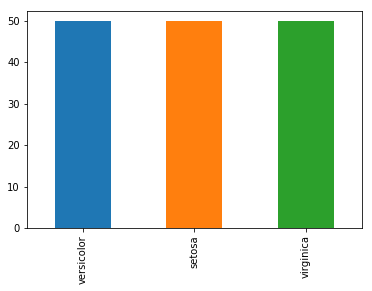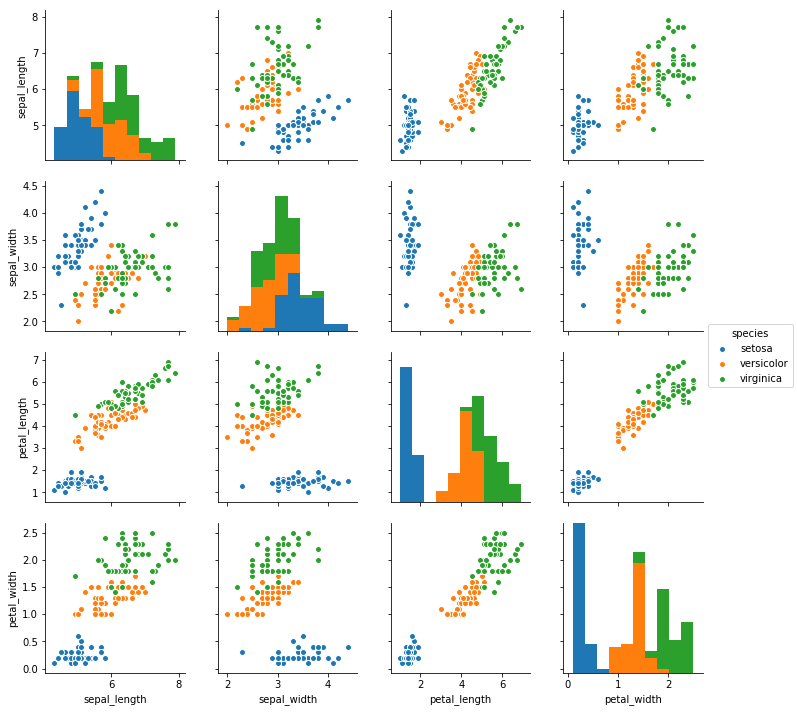# Naive Bayes classification using Scikit-Learn

## Naive Bayes classification - Sklearn¶¶

Naive Bayes classifier builds directly on conditional probability and this

$$p(y|x) = \frac{p(y \cap x)}{p(x)}$$

from the above formula, $p(y \cap x)$ can be written as

$$p(y \cap x) = p(x | y).p(y)$$

thus

$$p(y|x) = \frac{p(x|y).p(y)}{p(x)}$$

In machine learning, Naive Bayes is used to compute conditional probability of predicted class $y$ occuring given all the predictor variables $x$. In other words, Bayes theorem relates P(outcome/evidence) (what we want to predict) to P(evidence / outcome) (training set).

The algorithm builds the conditional probability and applies prediction. The algorithm is called naive because it assumes independence between predictor variables, while in reality this may not be true in all cases.

$$P(A_i | B_j) = \frac{P(B_j | A_i)P(A_i)}{P(B_j | A_1)P(A_1) + P(B_j | A_2)P(A_2) + … + P(B_j | A_k)P(A_k)}$$

Consider $A_1 ,… A_k$ as $k$ predictor variables in machine learning. The Naive Bayes classifier will build the conditional probabilities of $p(B_j|A_k)$ to later predict what would $p(A_i | B_j)$ be.

### Naive Bayes on Iris dataset¶¶

#### EDA¶¶

In :
import seaborn as sns

In :
iris.head()

Out:
sepal_length sepal_width petal_length petal_width species
0 5.1 3.5 1.4 0.2 setosa
1 4.9 3.0 1.4 0.2 setosa
2 4.7 3.2 1.3 0.2 setosa
3 4.6 3.1 1.5 0.2 setosa
4 5.0 3.6 1.4 0.2 setosa
In :
iris.shape

Out:
(150, 5)
In :
iris['species'].value_counts().plot(kind='bar')

Out:
<matplotlib.axes._subplots.AxesSubplot at 0x1a0d0e03c8>In :
%matplotlib inline
sns.pairplot(iris, hue='species')

Out:
<seaborn.axisgrid.PairGrid at 0x1a17a970b8>#### Create features and labels¶¶

In :
X_iris = iris.drop('species', axis=1)
X_iris.shape

Out:
(150, 4)
In :
y_iris = iris['species']
y_iris.shape

Out:
(150,)

X_iris is in caps as X is a vector of multiple features for each record, whereas y_iris is in small case as it is a scalar for each record.

In [ ]: